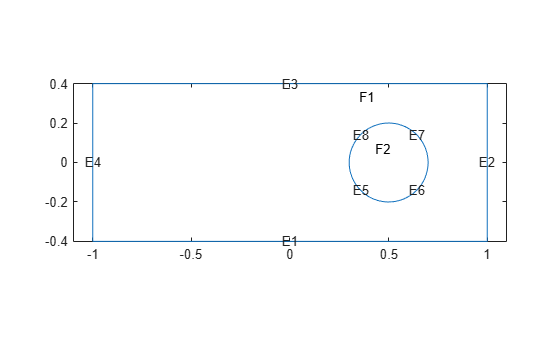# No Boundary Conditions Between Subdomains

There are two types of boundaries:

• Boundaries between the interior of the region and the exterior of the region

• Boundaries between subdomains - these are boundaries in the interior of the region

Boundary conditions, either Dirichlet or generalized Neumann, apply only to boundaries between the interior and exterior of the region. This is because the toolbox formulation uses the weak form of PDEs. See Finite Element Method Basics. In the weak formulation you do not specify boundary conditions between subdomains, even if coefficients are discontinuous between subdomains. So the toolbox does not support defining boundary conditions on subdomain boundaries.

For example, look at a rectangular region with a circular subdomain. The red numbers are the subdomain labels, the black numbers are the edge segment labels.

```% Rectangle is code 3, 4 sides, % followed by x-coordinates and then y-coordinates R1 = [3,4,-1,1,1,-1,-.4,-.4,.4,.4]'; % Circle is code 1, center (.5,0), radius .2 C1 = [1,.5,0,.2]'; % Pad C1 with zeros to enable concatenation with R1 C1 = [C1;zeros(length(R1)-length(C1),1)]; geom = [R1,C1]; % Names for the two geometric objects ns = (char('R1','C1'))'; % Set formula sf = 'R1 + C1'; % Create geometry gd = decsg(geom,sf,ns); % View geometry pdegplot(gd,'EdgeLabels','on', ... 'FaceLabels','on') xlim([-1.1 1.1]) axis equal```You need not give boundary conditions on segments 5, 6, 7, and 8, because these are subdomain boundaries, not exterior boundaries.

However, if the circle is a hole, meaning it is not part of the region, then you do give boundary conditions on segments 5, 6, 7, and 8.

## Support평가판 신청